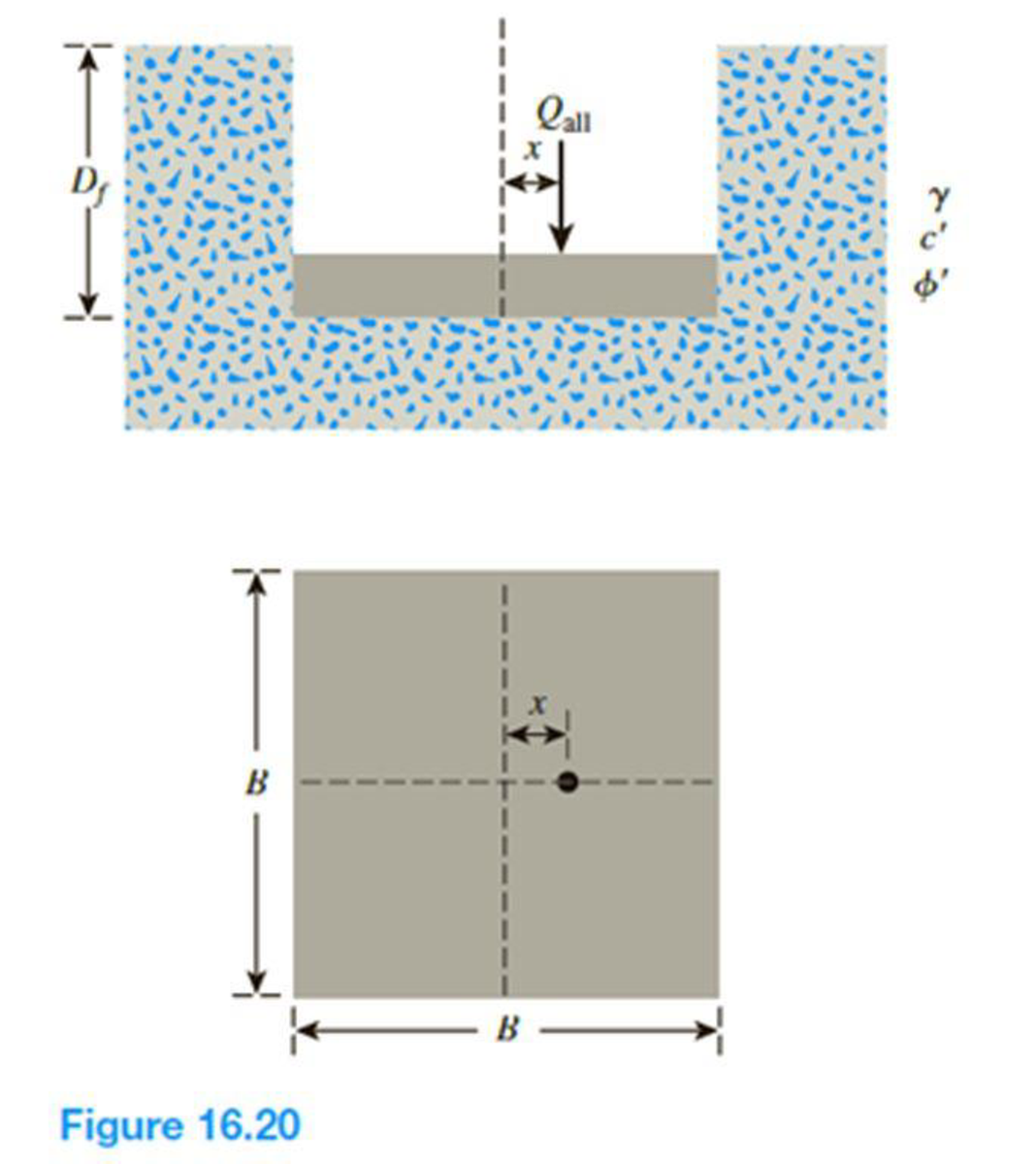Chapter 16, Problem 16.17PPrinciples of Geotechnical Enginee...

9th Edition
Braja M. Das + 1 other
ISBN: 9781305970939

Solutions

Chapter
SectionPrinciples of Geotechnical Enginee...

9th Edition
Braja M. Das + 1 other
ISBN: 9781305970939
Textbook Problem

A square footing on sand is subjected to an eccentric load as shown in Figure 16.20. Using Meyerhof’s effective area concept, determine the gross allowable load that the footing could carry with Fs = 4. Given: γ = 16 kN/m3, c′ = 0, ϕ′ = 29°, Df = 1.3 m, B = 1.75 m, and x = 0.25 m. Use Eqs. (16.32) through (16.42) for shape, depth, and inclination factors.Redo Problem 16.16 with the following data: γ = 118 lb/ft3, c′ = 700 lb/ft2, ϕ′ = 33°, Df = 4.5 ft, B = 6.25 ft, and x = 0.75 ft.

To determine

Find the gross allowable load that the footing could carry using the effective area concept.

Explanation

Given information:

The unit weight of the soil γ is 118lb/ft3.

The value of cohesion c is 700lb/ft2.

The soil friction angle ϕ is 33°.

The location of depth of footing base Df is 4.5 ft.

The width of the footing B is 6.25 ft.

The value of eccentricity x is 0.75 ft.

The factor of safety value Fs is 4.

Calculation:

Determine the effective width of the footing using the relation.

B=B2x

Substitute 6.25 ft for B and 0.75 ft for x.

B=6.252×0.75=4.75ft

The effective length of the footing is taken as the larger value of B(6.25ft) and B(4.75ft). Take the L as 6.25 ft.

Determine the shape factor λcs using the relation.

λcs=1+(BL)(NqNc)

Here, Nq and Nc are bearing-capacity factors.

Refer Table 16.2, “Bearing-capacity factors Nc, Nq, and Nγ” in the textbook.

For ϕ=33°;

The value of Nc is 38.64, Nq is 26.09, and Nγ is 35.19.

Substitute 4.75 ft for B, 6.25 ft for L, 26.09 for Nq, and 38.64 for Nc.

λcs=1+(4.756.25)(26.0938.64)=1.513

Determine the depth factor λcd using the relation.

λcd=1+0.4tan1(DfB)

Substitute 4.5 ft for Df and 4.75 ft for B.

λcd=1+0.4(4.54.75)=1.378

Determine the shape factor λqs using the relation.

λqs=1+(BL)tanϕ

Substitute 4.75 ft for B, 6.25 ft for L, and 33° for ϕ.

λqs=1+(4.756.25)tan33°=1.493

Determine the depth factor λqd using the relation.

λqd=1+2tanϕ(1sinϕ)2(DfB)

Substitute 33° for ϕ, 4.5 ft for Df, and 4.75 ft for B.

λqd=1+2tan33°(1sin33°)2(4

Still sussing out bartleby?

Check out a sample textbook solution.

See a sample solution

The Solution to Your Study Problems

Bartleby provides explanations to thousands of textbook problems written by our experts, many with advanced degrees!

Get Started

What is a relationship, and what three types of relationships exist?

Database Systems: Design, Implementation, & Management

What is Port Address Translation (PAT) and how does it work?

Principles of Information Security (MindTap Course List)

What does the term 3 Vs refer to?

Database Systems: Design, Implementation, & Management

Which type of hypervisor is installed on bare metal?

Network+ Guide to Networks (MindTap Course List)

In a typical database system, each department or area within an organization has its own set of files. (504)

Enhanced Discovering Computers 2017 (Shelly Cashman Series) (MindTap Course List)